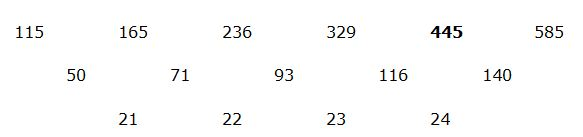# IBPS Clerk Prelims Quantitative Aptitude Questions 2019 (Day-07)

Dear Aspirants, Our IBPS Guide team is providing new series of Quantitative Aptitude Questions for IBPS Clerk Prelims 2019 so the aspirants can practice it on a daily basis. These questions are framed by our skilled experts after understanding your needs thoroughly. Aspirants can practice these new series questions daily to familiarize with the exact exam pattern and make your preparation effective.

Check here for IBPS Clerk Prelims Mock Test 2019

[WpProQuiz 7282]

wrong number series

Directions (1 – 5): Find out the wrong number in the following number series.

1) 102, 615, 3060, 12240, 36720

a) 615

b) 102

c) 3060

d) 36720

e) 12240

2) 613, 662, 695, 723, 739, 748

a) 748

b) 695

c) 723

d) 613

e) 739

3) 115, 165, 236, 329, 441, 585

a) 165

b) 236

c) 441

d) 115

e) 585

4) 18, 54, 135, 270, 405, 202.5

a) 270

b) 18

c) 135

d) 202.5

e) 54

5) 20, 21, 44, 135, 544, 2722

a) 44

b) 135

c) 544

d) 50

e) 2722

Data Interpretation

Directions (6 – 10): Study the following information carefully and answer the questions given below.

The following column chart shows the information about fee details for different type of vehicle to pass a toll gate.6) Find the average fee for all the vehicle for all type of the journey.

a) 432.5

b) 433.5

c) 216.25

d) 215.75

e) 469.25

7) The ratio of total fee collected in that toll gate for LCVs for single and multiple journeys is 30: 31. If 800 LCVs paid for multiple journeys, find the ratio of number of LCVs paid for single journey and LCVs paid for multiple journeys.

a) 1: 2

b) 3: 2

c) 2: 3

d) 4: 5

e) 2: 1

8) If a bus crosses the toll gate 5 times and pay for multiple journey one time, find the approximate profit percentage.

a) 120%

b) 70%

c) 240%

d) 340%

e) 140%

9) The fee for MAV for single and multiple journeys decreased and increased respectively by 10%. If the MAVs paid for single and multiple journeys are 637 and 351 respectively, find the ratio of fee paid for them.

a) 2: 1

b) 3: 1

c) 1: 2

d) 1: 1

e) 1: 3

10) Find the ratio of the fee paid for single and multiple journeys for all the vehicle together.

a) 139: 138

b) 205: 139

c) 139: 205

d) 207: 139

e) 139: 207

Directions (1-5):

102 * 6 = 612

612 * 5 = 3060

3060 * 4 = 12240

12240 * 3 = 36720

613 + 72 = 662

662 + 62 = 698

698 + 52 = 723

723 + 42 = 739

739 + 32 = 74818 * 3 = 54

54 * 2.5 = 135

135 * 2 = 270

270 * 1.5 = 405

405 * 1 = 405

20 * 1 + 1 = 21

21 * 2 + 2 = 44

44 * 3 + 3 = 135

135 * 4 + 4 = 544

544 * 5 + 5 = 2725

Directions (6-10):

Required average = (60 + 85 + 100 + 155 + 205 + 305 + 330 + 490)/4 = 432.5

Total fee LCVs paid for single journey = [(800 * 155)/31] * 30 = 120000

Number of LCVs paid for single journey = 120000/100 = 1200

Required ratio = 1200: 800 = 3: 2

The fee for single journey 5 times = 205 * 5 = 1025

Profit % = [(1025 – 305)/305] * 100 = 236% = 240%

The fee for MAV for single journey = 330 * 90/100 = 297

The fee for MAV for multiple journeys = 490 * 110/100 = 539

Required ratio = (297 * 637): (539 * 351) = 1: 1